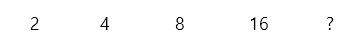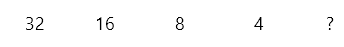# Number Sequences Explanation 2 - Exponential Series

#### Number sequences Explanation 2 - Exponential series

With an exponential relationship the steps between the numbers are increasing or decreasing. The simplest example of an exponential relationship is:Most people will see that the next number in the series if found by multiplying by 2, so the answer is 16 * 2 = 32. The same is true if we reverse the sequence:Answer: 4 : 2 = 2

We will first work out a number of examples and show you how to draw the arches. Then you can do 3 practice tasks yourself.